# Systems Biology · Quiz One

## Basic principle of mass spectrometry

### 1. History questions:

• Who invented mass spectrometer?
Joseph J. Thomson
• Who was the first one to win the Nobel Prize using mass spectrometry?
Joseph J. Thomson (1906 Physics, electron)
• Who were the ones that discovered two modern methods for ionization of biological molecules for biomedical applications by mass spectrometry?
John Fenn: ESI; Koichi Tanaka: MALDI

### 2. Here is a part of a mass spectrum of a peptide ion: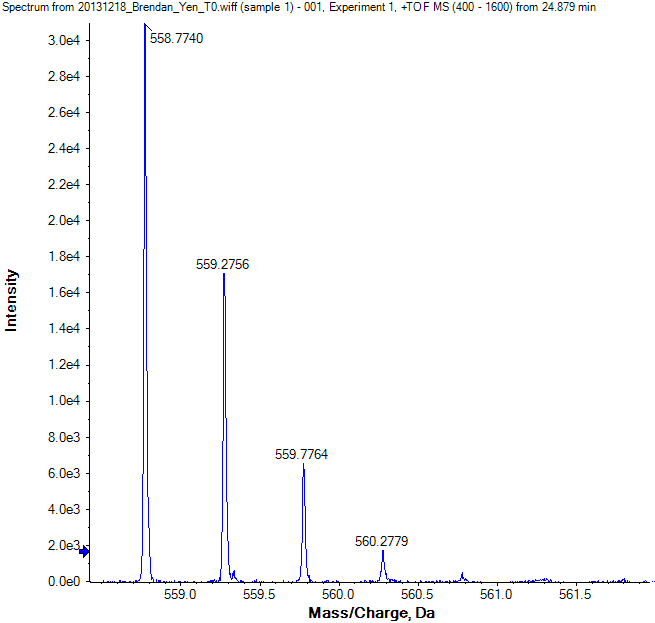• What is on the X-axial?
mass/charge ratio (m/z)
m = mass in Daltons (Da) or atomic mass units (u)
z = net positive or negative charge
• What is on the Y-axial?
the intensity of the ion signal at each particular m/z value
• Which peak is the monoisotopic ion?
The first peak labeled with 558.7740
• What is the peak labeled with 559.2756?
The average ion The [M+1] peak in Isotopic Ion Cluster (one atom altered)

### 3. Using the same spectrum above, please calculate the charge state of the peptide ion.

\begin{aligned} \Delta\left(\frac{m}{z}\right)&=\frac{\left|558.7740-559.2756\right|+\left|559.2756-559.7764\right|+\left|559.2779-560.2779\right|}{3}\\ &=\frac{560.2779-558.7740}{3}\\ &=0.5013\approx\frac{1}{2} \end{aligned}

### 4. What is the mass accuracy in ppm of the following peptide measurement (theoretical monoisotopic [M+H]+ = 1761.954)?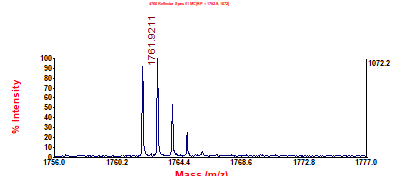$$ac={10}^6\times\frac{1761.954-1761.9211}{1761.954}=18.7ppm$$

### 5. Calculate the mass of the intact protein from its ESI charge envelope shown below.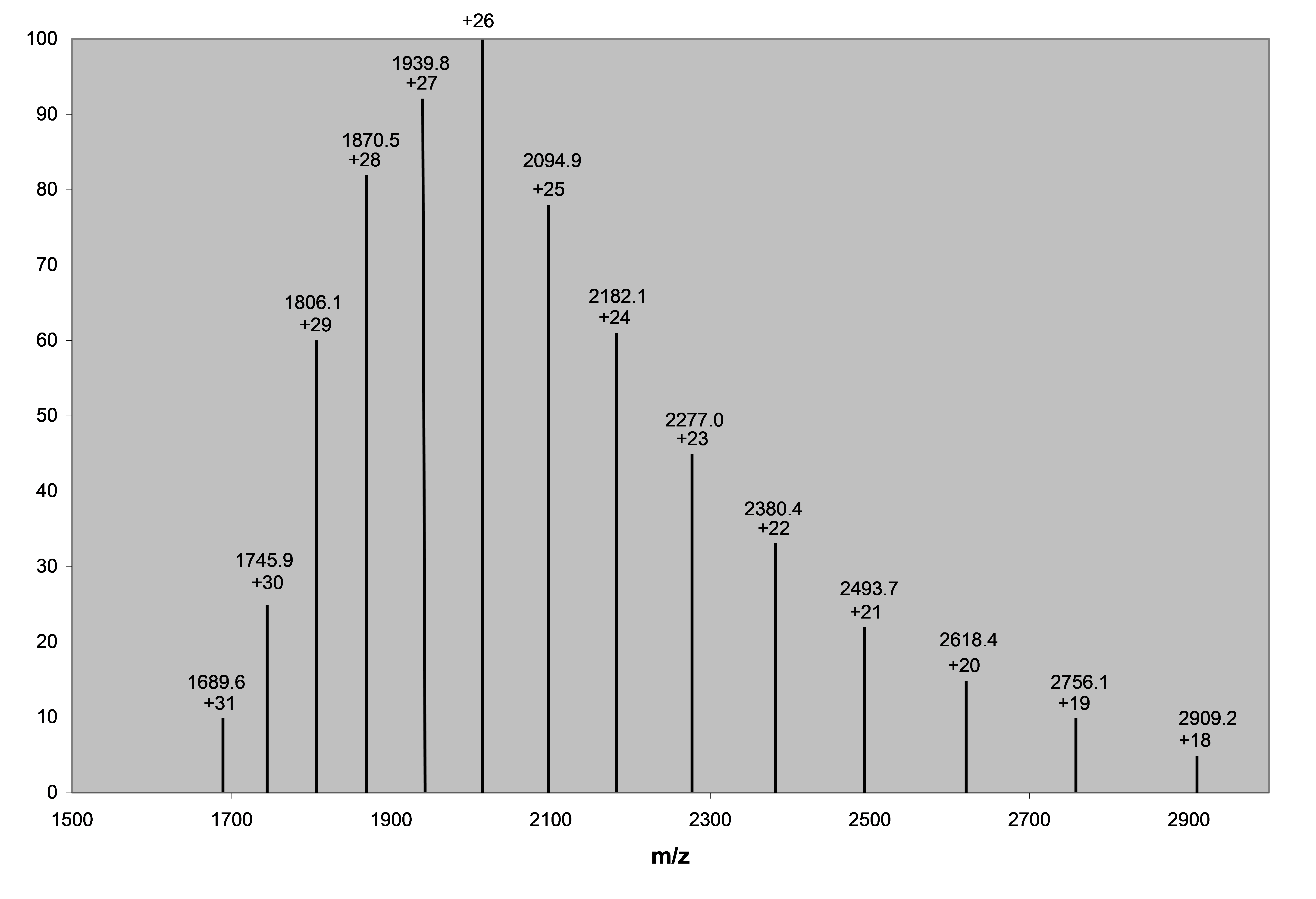Import data into matlab for fitting:
$$\frac{m}{z}=\frac{M}{n}+1$$$$x=n,y=\frac{m}{z}$$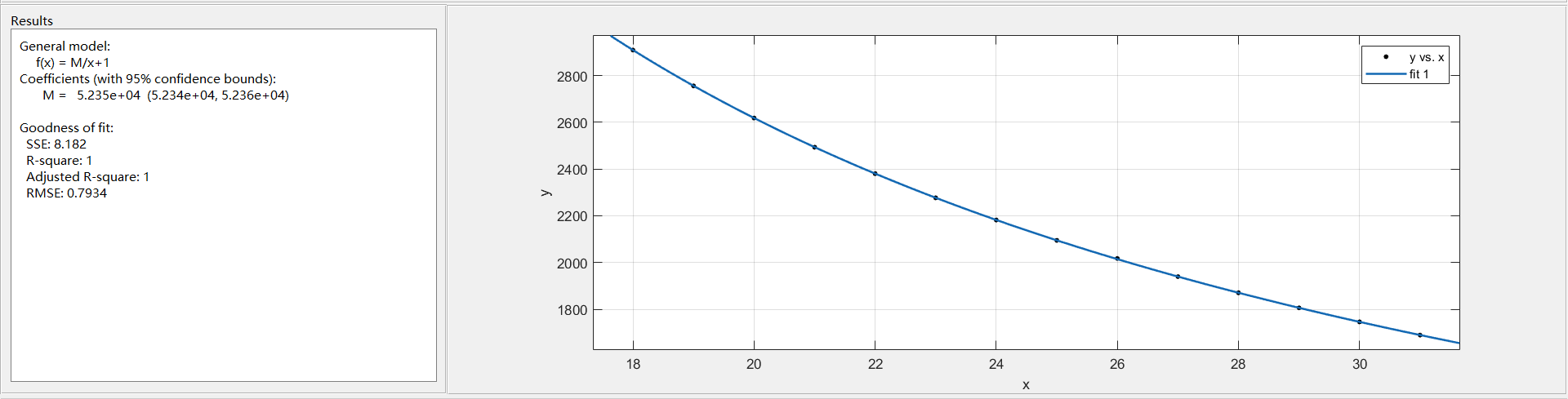$$M=52348$$

## Mass spectrometry: Instrumentation

### 1. What are the solvents for ESI? How to improve solvent evaporation in ESI?

Many solvents can be used in ESI and are chosen based on the solubility of the compound of interest, the volatility of the solvent and the solvent’s ability to donate a proton.

• Heat: 250-300℃
• Neutral gas flow (nitrogen)
• Vacuum
• Volatile organic solvents: Methanol/Acetonitrile

### 2. If you need to choose a compound as the matrix to analyze an intact protein in MALDI, which one do you need to choose?

UV light- absorbing weak organic acids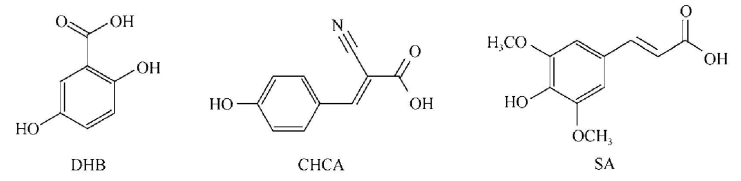### 3. What is the purpose of delayed extraction in MALDI?

To reduce spreading of the ions and differences in initial velocity and energy so that ions of the same m/z travel together through the mass spectrometer and give high resolution peaks.

### 4. There are many mass analyzers to choose from for a mass spectrometric analysis. Which factors do you need to consider for a mass analyzer?

• Speed(scan speed): how fast an analyzer can complete an analysis over a specified mass range
• Sensitivity: represented by the lowest concentration of sample that can be detected by the instrument
• Mass accuracy: the difference between the measured and theoretical masses of an ion
• Resolution: the ability of the instrument to distinguish between ions of different m/z
• Mass range: the range of m/z values that can be effectively separated by the mass analyzer

### 5. Can you list at least five mass analyzers? Separate them into low and high resolution groups.

high low
TOF
FT-ICR
Orbitrap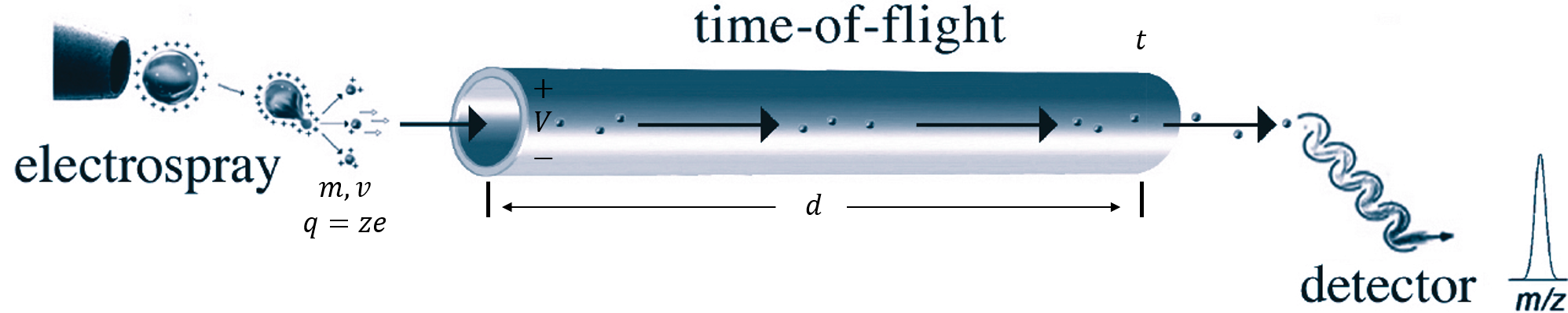$$\begin{cases} \frac{1}{2}mv^2=Vze\\ \frac{d}{t}=v \end{cases}$$
$${\Rightarrow}\frac{m}{z}=\frac{2Vet^2}{d^2}$$